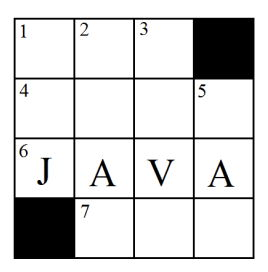시간 제한 메모리 제한 제출 정답 맞은 사람 정답 비율
2 초 512 MB 0 0 0 0.000%

문제

A crossword puzzle consists of a grid of squares in which intersecting words are placed, one letter per square. Some grid squares are filled black indicating that no letter should go there. Solvers are given clues for each word, and each clue is identified by a starting clue number and either the word Across (for horizontal words) or Down (for vertical words). Each square that can start a word is given a clue number, starting in the upper left and proceeding left-to-right, row-wise. A square will contain an across-clue number if the square to its left is either black or off the grid, and a square will contain a down-clue number if the square above it is either black or off the grid. See Figure E.1 for a simple example.

Will Longz is an avid crossword puzzle enthusiast and as with most solvers, he first tries to solve clues where some of the letters are already present (from already solved clues whose answers intersect the clue he is working on). Typically, the closer to the beginning of the word that these letters are, the better. Will has developed a metric to help him decide the order in which he should solve clues. If the answer to a clue spans n squares, he assigns the value n to the first of these squares, n − 1 to the second square, and so on ending with assigning 1 to last square. The rank of any unsolved clue is then defined as follows: for each square that already has a letter in it, add the value assigned to that square to a running total. Then divide this total by the sum of all the values assigned to that clue to get that clue’s rank. Once this is done for all the clues, Will solves the clue with the highest rank. If there is a tie, he selects an across clue over a down clue. If there is still a tie he picks the clue with the smallest starting clue number. After each clue is solved Will recalculates the rankings before selecting the next highest ranked clue.

As an example, consider the crossword in Figure E.1 where the 6-Across clue has already been solved (we’ll used 6A as a shorter specification for this clue). To determine the rank of the 1-Down (1D) clue, Will first assigns the values 3, 2 and 1 to each of the squares going down. Since the last square has a letter in it, the ranking of this clue is 1/(3 + 2 + 1) = 1/6. In a similar fashion he determines that the ranking of clues 2D and 3D are 2/10 and the ranking of 5D is 2/6. The rankings of the three unsolved across clues 1A, 4A and 7A are all 0. Thus the next clue to solve is 5D (we’ll be optimistic and always assume Will can solve any clue). Once this is solved, the rankings of 4A and 7A become 1/10 and 1/6, respectively. Since there is now a tie between the highest ranking clues (2D and 3D) and they are both down clues, Will picks the down clue with the lowest clue number and solves 2D. Proceeding similarly, the remaining clues are solved in the following order (with their ranking at time of solution in parentheses): 7A (4/6), 4A (4/10), 3D (6/10) and 1A (3/6). Note that clue 5D is not on the list, as it is completely solved by the previously solved clues (namely 4A, 6A and 7A).Figure E.1: Example crossword puzzle.

For this problem, you will be given a crossword puzzle grid with zero or more squares already filled in and you must determine the order in which unsolved clues should be solved. The filled-in squares may or may not correspond to completely solved clues. One final twist: we will only give you the black squares and letters in the puzzle. You must determine the clue numbers (I bet Will could do it!).

입력

Input starts with a line containing two integers r c (1 ≤ r, c ≤ 50) specifying the number of rows and columns in the crossword grid. Following this are r lines each containing c characters which describe the puzzle. Each of these characters is either a ‘.’ (indicating an empty square), a ‘#’ (indicating a black square), or an uppercase alphabetic English character (indicating a solved square). There will always be at least one empty square in the grid.

출력

Display the order in which clues should be solved one per line, using the metric described above. Each clue should start with the clue number followed by either the letter ‘A’ or ‘D’ (for an across or down clue).

4 4
...#
....
JAVA
#...

5D
2D
7A
4A
3D
1A